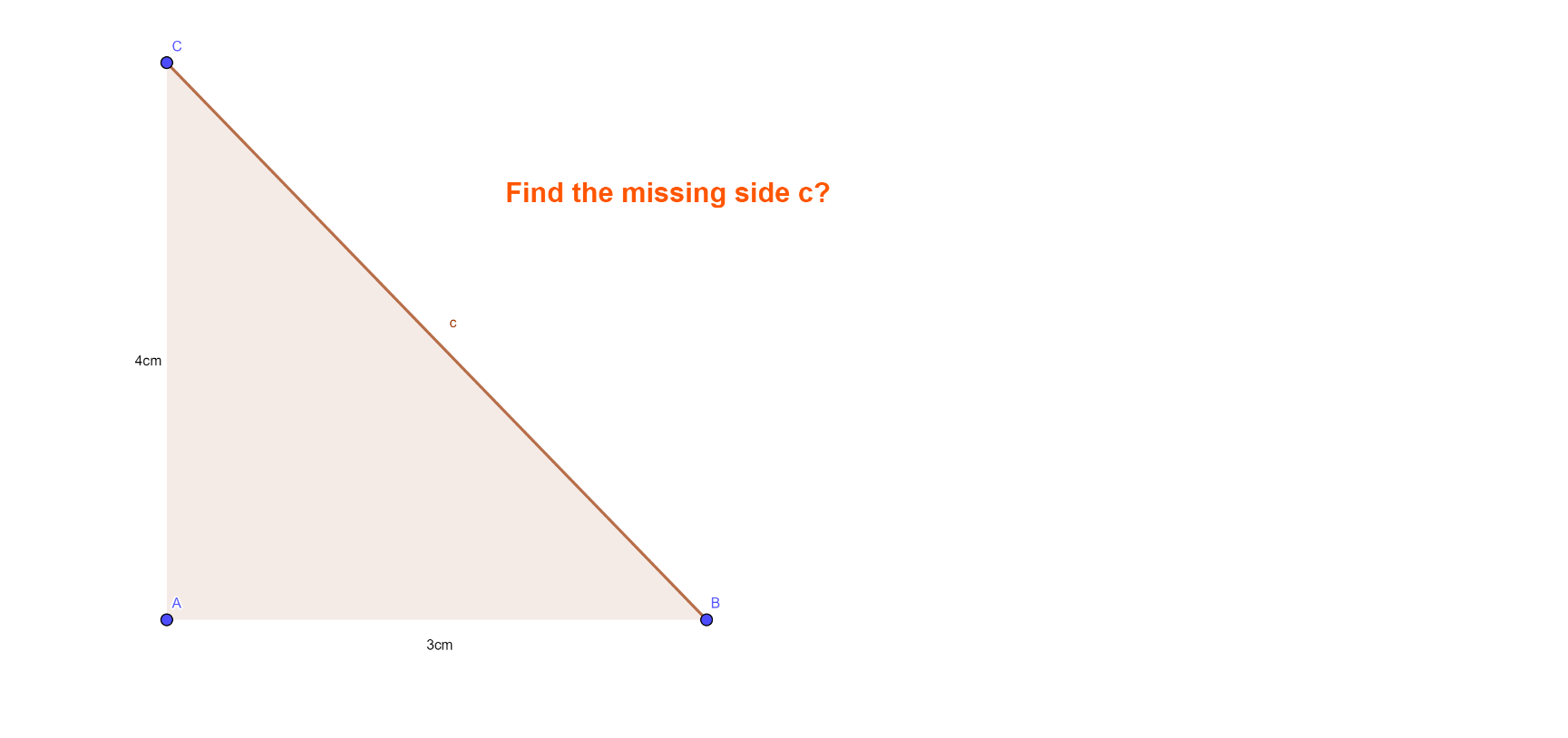# Pythagoras Theorem

## Move the points A, B or C and see how the values are changing.

A square is constructed on each side of the triangle. The area of each square is shown. Write down an expression for finding the side AC? Hint: Use the area.

Write down an expression to find the side AB

What is the side, c, called?

## Question: Find the missing side c in the triangle below?Question: Use the Pythagoras theorem to find the missing side, BCUse the Pythagoras theorem to find the missing side. Hint: This time its a short side we are trying to find, not the hypotenuse.

## Question: Find xQuestion: Find x